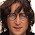# LCM and HCF tricks, problems and formulas

LCM i.e. least common multiple is a number which is multiple of two or more than two numbers. For example: The common multiples of 3 and 4 are 12,24 and so on. Therefore, l.c.m.is smallest positive number that is multiple of both. Here, l.c.m. is 12.. HCF i.e. highest common factor are those integral values of number that can divide that number. LCM and HCF problems are very important part of all competitive exams.

## Some important l.c.m. and h.c.f. tricks:

1) Product of two numbers = Their h.c.f. * Their l.c.m.

2) h.c.f. of given numbers always divides their l.c.m.

3) h.c.f. of given fractions =     h.c.f. of numerator
l.c.m. of denominator

4) l.c.m. of given fractions =    l.c.m. of numerator
h.c.f. of denominator

5) If d is the h.c.f. of two positive integer a and b, then there exist unique integer m and n, such that
d = am + bn

6) If p is prime and a,b are any integer then ,This implies   P or P
ab                       a      b

7) h.c.f. of a given number always divides its l.c.m.

## Most important points about l.c.m. and h.c.f. problems :

1) Largest number which divides x,y,z to leave same remainder = h.c.f. of y-x, z-y, z-x.

2) Largest number which divides x,y,z to leave remainder R (i.e. same) = h.c.f of x-R, y-R, z-R.

3) Largest number which divides x,y,z to leave same remainder a,b,c  = h.c.f. of x-a, y-b, z-c.

4) Least number which when divided by x,y,z and leaves a remainder R in each case = ( l.c.m. of x,y,z) + R

## HCF and LCM questions:

Problem 1: Least number which when divided by 35,45,55 and leaves remainder 18,28,38; is?
Solution: i) In this case we will evaluate l.c.m.
ii) Here the difference between every divisor and remainder is same i.e. 17.
Therefore, required number = l.c.m. of (35,45,55)-17 = (3465-17)= 3448.

Problem 2: Least number which when divided by 5,6,7,8 and leaves remainder 3, but when divided by 9, leaves no remainder?
Solution: l.c.m. of 5,6,7,8 = 840
Required number = 840 k + 3
Least value of k for which (840 k + 3) is divided by 9 is 2
Therefore, required number = 840*2 + 3
= 1683

Problem 3: Greater number of 4 digits which is divisible by each one of 12,18,21 and 28 is?
Solution: l.c.m. of 12,18,21,28 = 254
Therefore, required number must be divisible by 254.
Greatest four digit number = 9999
On dividing 9999 by 252, remainder = 171
Therefore, 9999-171 = 9828.#### What's trending in BankExamsToday

Smart Prep Kit for Banking Exams by Ramandeep Singh - Download here1.plz send me word list on jaspreet_preeti@ymail.com ..........

2.It is a Trial and Error method.
When yo take K = 1, 843 is not divisible by 9
but when we take K = 2, 1683 is divisible by 9.

3.can u plz explain how did u get value of k=2 in problem no. 2

4.5.hey sir , there is contradiction between MOST IMP pt 4 nd EXAMPLE 1.......+R OR -R ????
IN POINT 4 YOU ARE SAYING +R bt in EXAMPLE -R ???????

6.YES sir ,, i conform .././.

1.you better check before publishing wrong things like............
4) Least number which when divided by x,y,z and leaves a remainder R in each case = ( l.c.m. of x,y,z)+ R

7.Hey Guys, (Anne and Akhilesh)
I Guess there's some confusion.... that asked you to put these comments.. Raman is Right here..

There are two points:
1. The least number, which when divided by x,y and z leaves the remainder a,b and c respectively
= [LCM of (x,y,z)] - k
where k = (x-a) = (y-b) = (z-c)

2. The least number, which when divided by x,y and z leaves the same remainder r in each case
= [LCM of (x,y,z)] + r

Understand the difference between two. One is having different remainders another is having same remainder.

Thanks for commenting. Follow us on Telegram. Search BankExamsToday